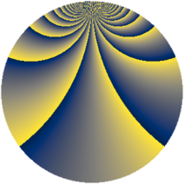# Properties

 Label 2760.2.doLevel $2760$ Weight $2$ Character orbit 2760.do Rep. character $\chi_{2760}(233,\cdot)$ Character field $\Q(\zeta_{44})$ Dimension $2880$ Sturm bound $1152$

# Related objects

## Defining parameters

 Level: $$N$$ $$=$$ $$2760 = 2^{3} \cdot 3 \cdot 5 \cdot 23$$ Weight: $$k$$ $$=$$ $$2$$ Character orbit: $$[\chi]$$ $$=$$ 2760.do (of order $$44$$ and degree $$20$$) Character conductor: $$\operatorname{cond}(\chi)$$ $$=$$ $$345$$ Character field: $$\Q(\zeta_{44})$$ Sturm bound: $$1152$$

## Dimensions

The following table gives the dimensions of various subspaces of $$M_{2}(2760, [\chi])$$.

Total New Old
Modular forms 11840 2880 8960
Cusp forms 11200 2880 8320
Eisenstein series 640 0 640

## Trace form

 $$2880q + O(q^{10})$$ $$2880q + 24q^{15} + 32q^{21} + 16q^{25} + 24q^{27} + 16q^{31} - 72q^{37} - 48q^{55} - 48q^{57} - 48q^{61} - 16q^{63} - 80q^{67} - 16q^{73} + 108q^{75} + 56q^{81} - 16q^{85} + 40q^{87} + 64q^{91} + 72q^{93} + O(q^{100})$$

## Decomposition of $$S_{2}^{\mathrm{new}}(2760, [\chi])$$ into newform subspaces

The newforms in this space have not yet been added to the LMFDB.

## Decomposition of $$S_{2}^{\mathrm{old}}(2760, [\chi])$$ into lower level spaces

$$S_{2}^{\mathrm{old}}(2760, [\chi]) \cong$$ $$S_{2}^{\mathrm{new}}(345, [\chi])$$$$^{\oplus 4}$$$$\oplus$$$$S_{2}^{\mathrm{new}}(690, [\chi])$$$$^{\oplus 3}$$$$\oplus$$$$S_{2}^{\mathrm{new}}(1380, [\chi])$$$$^{\oplus 2}$$